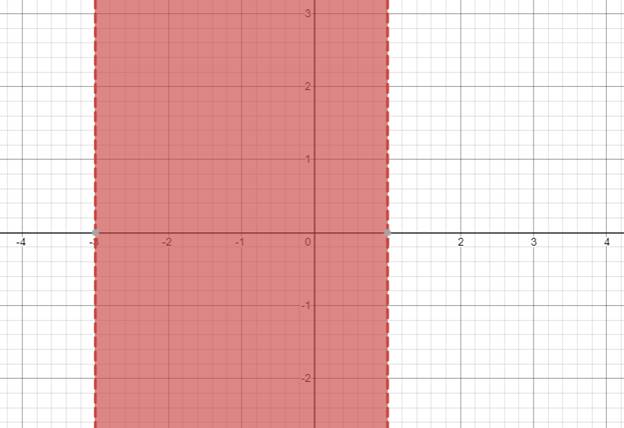# The solution of given nonlinear inequality equation and plot a graph for it.### Precalculus: Mathematics for Calcu...

6th Edition
Stewart + 5 others
Publisher: Cengage Learning
ISBN: 9780840068071### Precalculus: Mathematics for Calcu...

6th Edition
Stewart + 5 others
Publisher: Cengage Learning
ISBN: 9780840068071

#### Solutions

Chapter 1.7, Problem 46E
To determine

## The solution of given nonlinear inequality equation and plot a graph for it.

Expert Solution

3<x<1

### Explanation of Solution

Given:

Non-linear inequality equation

x2+2x>3

Calculation,

Non-linear inequality equation,

x2+2x>3x2+2x3>0x2+(31)x3>0x2+3xx3>0x(x+3)1(x+3)>0(x1)(x+3)>0Now,x+3>0x>3andx1>0x<1so,3<x<1

Now, graph of x2+2x>3Conclusion:

Hence, the values of x lies between the -3 and 1.

### Have a homework question?

Subscribe to bartleby learn! Ask subject matter experts 30 homework questions each month. Plus, you’ll have access to millions of step-by-step textbook answers!